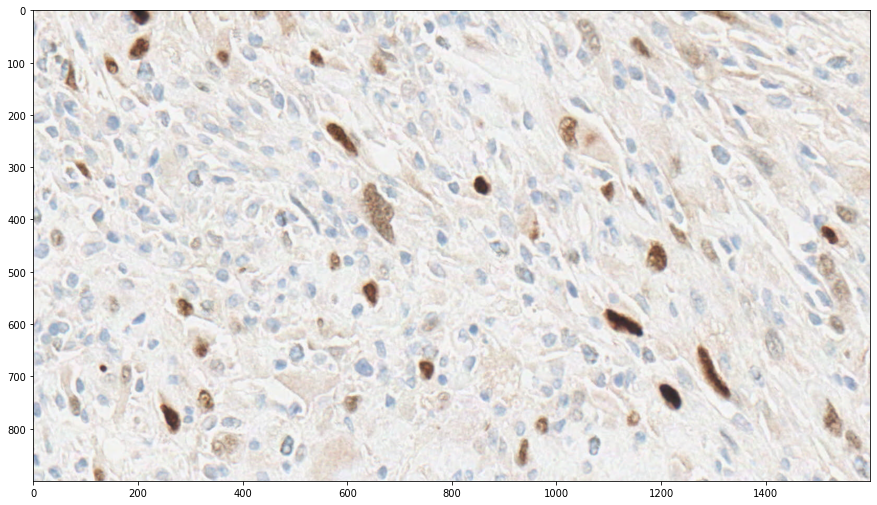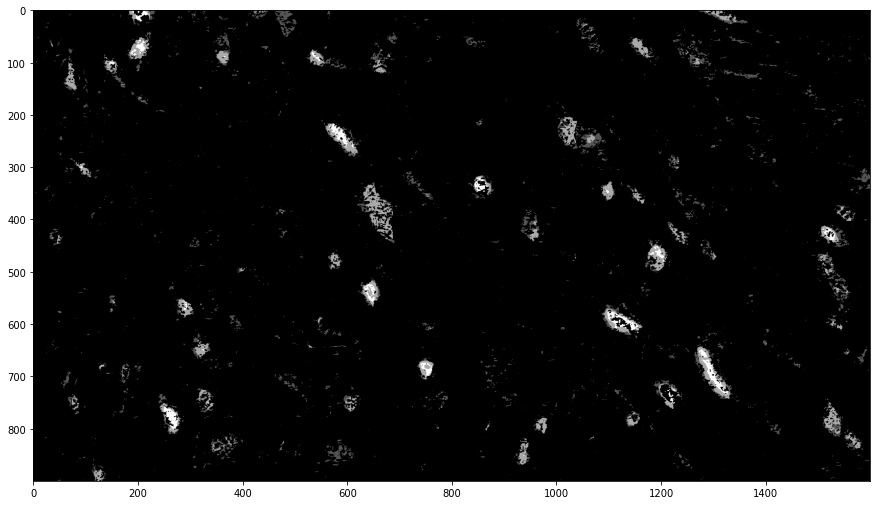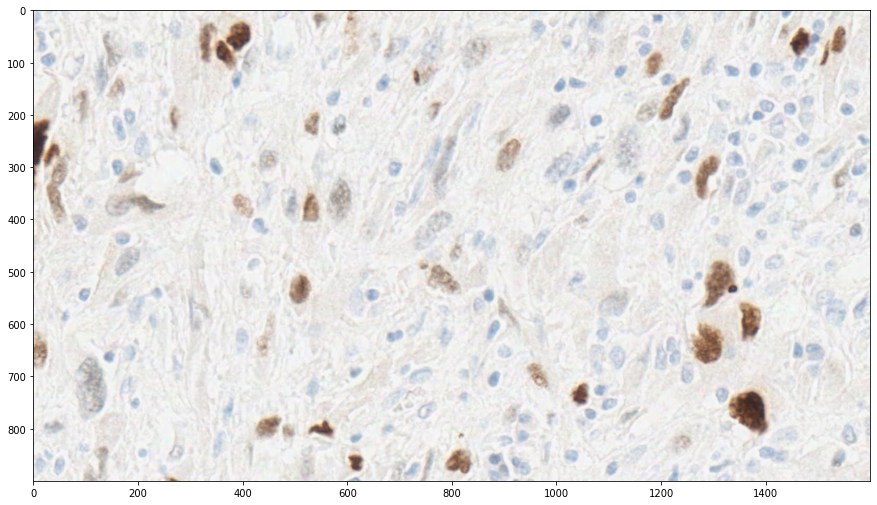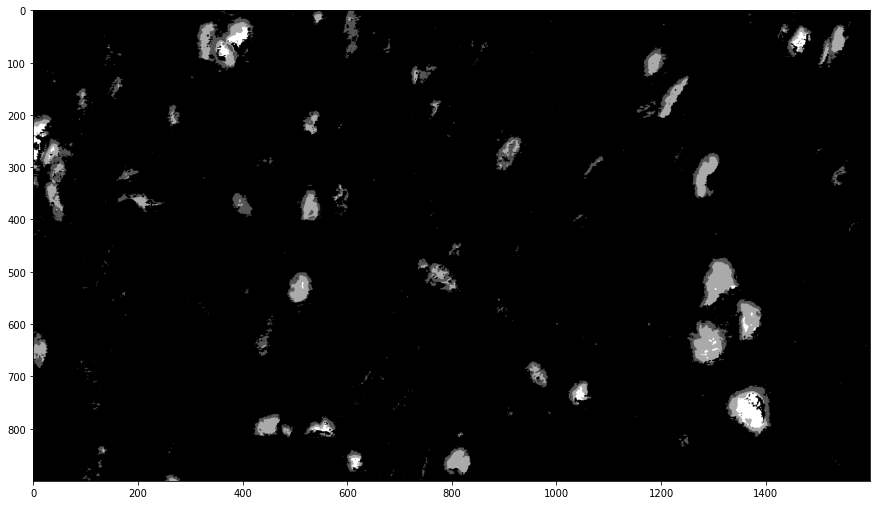# Positive pixel count and parallel processing with Dask¶

## Overview¶

Positive pixel count is a routine that classifies pixels by their position in HSI Color Space and computes statistics based on this classification.

HistomicsTK has two main functions for positive pixel counting, count_slide and count_image, both of which live in the histomicstk.segmentation.positive_pixel_count module (imported in this notebook as simply ppc). count_image operates on an in-memory image in a format compatible with NumPy’s ndarray type, and returns both the classification statistics and a label image that may be useful for visualization or further analysis.

count_slide accepts a path to an image instead, and while it can also carry out exactly the same computation as count_image, its advantage lies in its ability to distribute its computation using Dask and to operate on images too large to fit in memory. This ability comes at a cost – to enable it, the generation of the label image must be disabled. (For the curious, the necessary underlying support for writing large images a tile at a time is lacking.) The HistomicsTK CLI PositivePixelCount is a wrapper around this function.

The rest of this example is subdivided, in order, into count_image and count_slide examples, followed by a CLI example.

:

# Configuration and imports of other libraries
from __future__ import print_function

import large_image
%matplotlib inline
import matplotlib.pyplot as plt
import skimage.io

#Some nice default configuration for plots
plt.rcParams['figure.figsize'] = 15, 15
plt.rcParams['image.cmap'] = 'gray'

# Import and alias positive_pixel_count
import histomicstk.segmentation.positive_pixel_count as ppc


## Analyzing a small image with count_image¶

We start with count_image. The image we will look at is small and can be analyzed quickly.

:

image_url = ('https://data.kitware.com/api/v1/file/'

print('Input image')
plt.imshow(im_input)
plt.show()

Input imageWith a helper function, it becomes reasonable to use count_image interactively to explore parameter values. A more advanced analysis might also look at the HSI values directly.

Here, we show briefly how one can use the helper function count_and_label defined below.

:

def count_and_label(params):
"Compute the label image with count_image, and then display it"
label_image = ppc.count_image(im_input, params)
plt.imshow(label_image)
plt.show()


To start out, we’ll pick a set of parameters. The HSI color space used by the routines is defined to use values in the range [0,1].

:

import ipywidgets as widgets
from ipywidgets import interactive

params= {
'hue_value': widgets.FloatSlider(min=0.0,max=1.0,step=0.004,style = {'description_width': 'initial'}, value=0.05),
'hue_width':widgets.FloatSlider(min=0.0,max=1.0,step=0.05,style = {'description_width': 'initial'}, value=0.15),
'saturation_minimum': widgets.FloatSlider(min=0.0,max=1.0,step=0.05,style = {'description_width': 'initial'}, value=0.05),
'intensity_upper_limit': widgets.FloatSlider(min=0.0,max=1.0,step=0.05,style = {'description_width': 'initial'}, value=0.95),
'intensity_weak_threshold': widgets.FloatSlider(min=0.0,max=1.0,step=0.05,style = {'description_width': 'initial'}, value=0.65),
'intensity_strong_threshold':widgets.FloatSlider(min=0.0,max=1.0,step=0.05,style = {'description_width': 'initial'}, value=0.35),
'intensity_lower_limit':widgets.FloatSlider(min=0.0,max=1.0,step=0.05,style = {'description_width': 'initial'}, value=0.05)
}

def g(**params):
count_and_label(ppc.Parameters(**params))

w = interactive(g,**params)
display(w)

template_params = ppc.Parameters(**w.kwargs)


Internally, the label values are conveniently increasing, regularly spaced integers. Black is therefore background / negative, dark gray is weak positive, light gray is positive, and white is strong positive. To use the label values programmatically, use of the static attributes of ppc.Labels.NEGATIVE, .WEAK, .PLAIN, and .STRONG – is recommended.

This does a reasonably good job aleady, but let’s see what happens if we shift the hue center a bit. Since Parameters is a collections.namedtuple, we can use its ._replace method to substitute a value.

:

count_and_label(template_params._replace(hue_value=0.0))Here, we see that a number of pixels previously considered positive are now considered negative, creating holes in visible nuclei. This indicates that we’ve moved the hue range too far.

In any case, we can also view the statistics. Here we use the original parameter values.

:

stats, label_image = ppc.count_image(im_input, template_params)

def pp_namedtuple(t):
"Pretty-print a namedtuple by printing each field on its own line and left-aligning all values"
print(type(t).__name__)
maxlen = max(map(len, t._fields))
for f in t._fields:
print(f, getattr(t, f), sep=':' + ' ' * (maxlen - len(f)) + '\t')

pp_namedtuple(stats)

Output
NumberWeakPositive:                     62163
NumberPositive:                         24459
NumberStrongPositive:                   4948
NumberTotalPixels:                      1440000
IntensitySumWeakPositive:               49554.65359477124
IntensitySumPositive:                   12907.145098039215
IntensitySumStrongPositive:             1423.4183006535948
IntensityAverage:                       0.6976653597626302
RatioStrongToTotal:                     0.054035164355138145
IntensityAverageWeakAndPositive:        0.7210846977997558
RatioStrongToPixels:                    0.003436111111111111
RatioWeakToPixels:                      0.04316875
RatioTotalToPixels:                     0.06359027777777777


## Analyzing a large image with count_slide¶

count_slide has several more parameters than count_image. Besides make_label_image, which controls the creation of the label image as mentioned, there is also region, which instructs count_slide to operate on only the specified region of the image.

We will visualize its operation on a small region, and then run it on a larger region.

:

# Comment this out (or just don't run it) once you have the file

  % Total    % Received % Xferd  Average Speed   Time    Time     Time  Current
100 1668M  100 1668M    0     0  1955k      0  0:14:33  0:14:33 --:--:-- 1665k0  0:14:48  0:00:20  0:14:28 1975k  0  0:14:34  0:03:30  0:11:04 2128k     0  0:14:04  0:06:59  0:07:05 2154k18  0:00:14 1926k
curl: Saved to filename 'TCGA-DX-A6BG-01Z-00-DX2.34763958-0613-4069-9ACC-13D6633FE415.svs'

:

# Set up a basic configuration.  Change as needed.


:


### Client

Client-80fcf7a4-e10a-11ec-ac62-3529764f914a

 Connection method: Cluster object Cluster type: distributed.LocalCluster Dashboard: http://127.0.0.1:8787/status

### count_slide on a small region¶

Now we can inspect the image. We’ll first run the calculation to produce a label image, and then run it again without, allowing parallelization.

:

slide_path = 'TCGA-DX-A6BG-01Z-00-DX2.34763958-0613-4069-9ACC-13D6633FE415.svs'
region = dict(
left=50000, top=35000,
width=1600, height=900,
)

ts = large_image.getTileSource(slide_path)
im_region = ts.getRegion(region=region, format=large_image.tilesource.TILE_FORMAT_NUMPY)

print("The region")
plt.imshow(im_region)
plt.show()

The regionWe’ll reuse the parameters from before.

:

stats, label_image = ppc.count_slide(slide_path, template_params, region=region, make_label_image=True)
pp_namedtuple(stats)
plt.imshow(label_image)
plt.show()

Output
NumberWeakPositive:                     31591
NumberPositive:                         26850
NumberStrongPositive:                   5582
NumberTotalPixels:                      1440000
IntensitySumWeakPositive:               24633.738562091505
IntensitySumPositive:                   13946.745098039215
IntensitySumStrongPositive:             1549.1424836601307
IntensityAverage:                       0.626800152192038
RatioStrongToTotal:                     0.08718741702200772
IntensityAverageWeakAndPositive:        0.6601612508364114
RatioStrongToPixels:                    0.003876388888888889
RatioWeakToPixels:                      0.021938194444444444
RatioTotalToPixels:                     0.04446041666666667The output is about the same quality as before. As the small region used in the previous section is in fact extracted from another part of this slide, this is not too surprising.

We’ll now rerun for only the stats, which will make use of Dask. make_label_image defaults to False, so we simply omit it here. For the purposes of illustration, we force tile_grouping to 1 to process each tile in its own task. A larger value, 256, is used as the default value to reduce the overhead associated with Dask tasks.

:

# Note that we still return a tuple, though it now has length 1.
stats_dask, = ppc.count_slide(slide_path, template_params, region=region, tile_grouping=1)

Output
NumberWeakPositive:                     31591
NumberPositive:                         26850
NumberStrongPositive:                   5582
NumberTotalPixels:                      1440000
IntensitySumWeakPositive:               24633.73856209151
IntensitySumPositive:                   13946.745098039215
IntensitySumStrongPositive:             1549.142483660131
IntensityAverage:                       0.6268001521920381
RatioStrongToTotal:                     0.08718741702200772
IntensityAverageWeakAndPositive:        0.6601612508364115
RatioStrongToPixels:                    0.003876388888888889
RatioWeakToPixels:                      0.021938194444444444
RatioTotalToPixels:                     0.04446041666666667


The results are identical up to a tiny amount of floating point error, as can be seen below:

:

print("stats_dask - stats:")
pp_namedtuple(ppc.Output(**{f: getattr(stats_dask, f) - getattr(stats, f) for f in ppc.Output._fields}))

stats_dask - stats:
Output
NumberWeakPositive:                     0
NumberPositive:                         0
NumberStrongPositive:                   0
NumberTotalPixels:                      0
IntensitySumWeakPositive:               3.637978807091713e-12
IntensitySumPositive:                   0.0
IntensitySumStrongPositive:             2.2737367544323206e-13
IntensityAverage:                       1.1102230246251565e-16
RatioStrongToTotal:                     0.0
IntensityAverageWeakAndPositive:        1.1102230246251565e-16
RatioStrongToPixels:                    0.0
RatioWeakToPixels:                      0.0
RatioTotalToPixels:                     0.0


### count_slide on a large region¶

As a better exhibition of count_slide’s use of parallelism in computing statistics, we run it here on a much larger region, 30Kx30K pixels.

(By leaving out the region parameter, count_slide will look at the entire image. At 146Kx79K pixels, this image will cause count_slide to use a lot of memory, potentially enough to require swap space, without additional configuration of large_image’s caching behavior.)

:

large_region = dict(
left=60e3, top=30e3,
width=30e3, height=30e3,
)

stats, = %time ppc.count_slide(slide_path, template_params, large_region)

pp_namedtuple(stats)

CPU times: user 992 ms, sys: 87 ms, total: 1.08 s
Wall time: 16.1 s
Output
NumberWeakPositive:                     8017246
NumberPositive:                         4348294
NumberStrongPositive:                   599706
NumberTotalPixels:                      900000000
IntensitySumWeakPositive:               6295544.718954247
IntensitySumPositive:                   2316964.7856209157
IntensitySumStrongPositive:             174806.2339869282
IntensityAverage:                       0.6777592757254349
RatioStrongToTotal:                     0.04625488787486177
IntensityAverageWeakAndPositive:        0.6964927940530833
RatioStrongToPixels:                    0.00066634
RatioWeakToPixels:                      0.008908051111111112
RatioTotalToPixels:                     0.014405828888888889

:

# pp_namedtuple(template_params)
# print('\nRegion:', region)

:

# %%script sh
# # Stats and label image output must be specified via file arguments to
# # --returnparameterfile and --outputLabelImage, respectively.
# python ../../server/PositivePixelCount/PositivePixelCount.py \
#     'TCGA-DX-A6BG-01Z-00-DX2.34763958-0613-4069-9ACC-13D6633FE415.svs' \
#     0.05 0.15 0.05 0.95 0.65 0.35 0.05 --region 50000,35000,1600,900 \
#     --returnparameterfile stats.txt --outputLabelImage labelImage.png 2>/dev/null

:

# print("stats.txt:")
# for l in open('stats.txt'):
#     print(l.rstrip())

# print("\nlabelImage.png:")